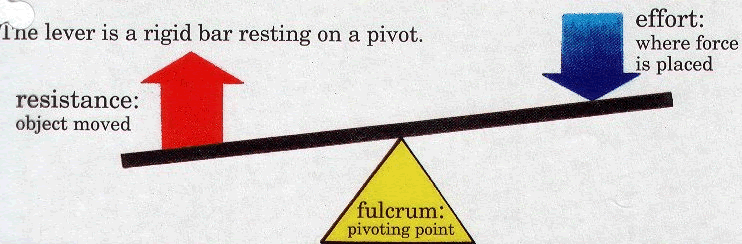TORQUE

WHAT IS TORQUE?

Torque is a measure of how much a force acting on an object causes that object to rotate. The object rotates about an axis, which we will call the pivot point (fulcrum*). We will call the force 'F'. The distance from the pivot point to the point where the force acts is called the lever arm, and is denoted by 'r'.  Torque is particularly important to understand as it relates to hosin sul (joint locks, releases, take downs, throws).

Torque = r x F

F = Force

r = Distance from pivot point to point where the force actsImagine pushing a door to open it. The force of your push (F) causes the door to rotate about its hinges (the pivot point, O). How hard you need to push depends on the distance you are from the hinges (r) (and several other things, but let's ignore them now). The closer you are to the hinges (i.e. the smaller r is), the harder it is to push. This is what happens when you try to push open a door on the wrong side. The torque you created on the door is smaller than it would have been had you pushed the correct side (away from its hinges).

Another other example is applying force to a wrench.  The longer the handle, the greater the distance from the pivot point (where the nut is located), so the greater the torque will be. This same idea applies to many moves in Taekwon Do, such as an armbar.  The torque is greater if you apply pressure at the wrist, then at the upper forearm, because of an increased distance from the pivot point (which in this case is the shoulder joint).

Note that a force applied at the pivot point will cause no torque since the moment arm would be zero (r = 0).

*The pivot point and fulcrum are basically synonymous. The pivot and fulcrum are the points of rotation of a lever. The fulcrum is more of a point against which lever is placed or on which it turns or is supported. The pivot, on the other hand, is an object (short shaft or pin) on which something turns or oscillates.